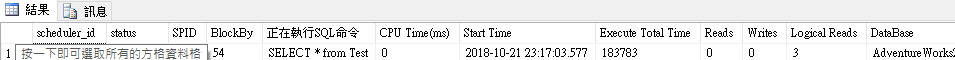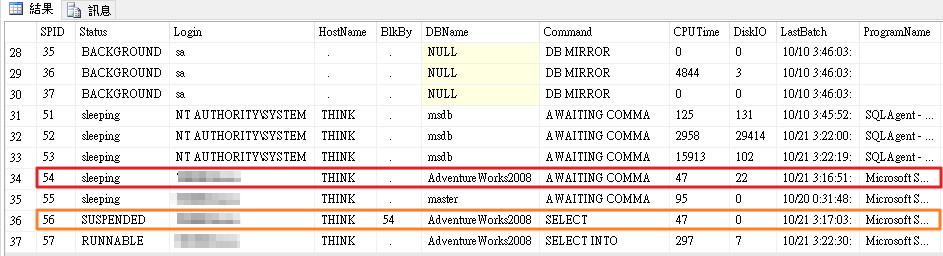2019 iT 邦幫忙鐵人賽DAY 21
1

SQL Server常見問題之一

--先建立測試用表格
CREATE TABLE Test
(ID INT IDENTITY,Name VARCHAR(20))
--塞入測試資料
INSERT INTO Test VALUES('1111'),('2222'),('3333'),('4444'),('5555')

--接著開啓交易後不要Commite!
BEGIN TRAN
UPDATE Test
SET NAME = 'testtest'
WHERE ID = 5

SELECT * from Test

--找鎖定的物件、類型以及被誰Blocking
SELECT      r.scheduler_id ,
status         ,
r.session_id   as SPID,
r.blocking_session_id as BlockBy,
substring(
ltrim(q.text),
r.statement_start_offset/2+1,
(CASE
WHEN r.statement_end_offset = -1
THEN LEN(CONVERT(nvarchar(MAX), q.text)) * 2
ELSE r.statement_end_offset
END - r.statement_start_offset)/2)
AS [正在執行SQL命令],
r.cpu_time      as [CPU Time(ms)],
r.start_time    as [Start Time],
r.total_elapsed_time as [Execute Total Time],
r.writes             as [Writes],
-- q.text, /* 完整的 T-SQL 指令碼 */
d.name               as [DataBase]
FROM        sys.dm_exec_requests r
CROSS APPLY sys.dm_exec_sql_text(sql_handle) AS q
LEFT JOIN sys.databases d ON (r.database_id=d.database_id)
WHERE       r.session_id > 50 AND r.session_id <> @@SPID
ORDER BY    r.total_elapsed_time descIT人員面面觀28